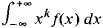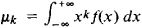# moment

(redirected from not for one moment)
Also found in: Dictionary, Thesaurus, Medical, Idioms.

## moment,

in physics and engineering, term designating the product of a quantity and a distance (or some power of the distance) to some point associated with that quantity. The most theoretically useful moments are moments of masses, areas, lines, and forces, including magnetic force. The concept of torque (propensity to turn about a point) is the moment of force. If a force tends to rotate a body about some point, then the moment, or turning effect, is the product of the force and the distance from the point to the direction of the force. The application of this concept is illustrated by pushing open a door: the farther from the hinge the push is applied, the less force is required. The principle of the moment of a force is perhaps best seen in the use of a leverlever,
simple machine consisting of a bar supported at some stationary point along its length and used to overcome resistance at a second point by application of force at a third point. The stationary point of a lever is known as its fulcrum.
. Extensions of this concept are important in mechanics, in topics such as inertia, center of gravity, equilibrium, and stability of structures, and in architectural problems. The moment of inertia of a body about a point is the sum, for each particle in the body, of the mass of the particle and the square of its distance from the point. The angular momentum of a body about a fixed axis is equal to the product of the momentum and the length of the moment arm (distance from the body to the axis). A torque acting on a rigid body acts to change its angular momentum by producing an angular acceleration.
The following article is from The Great Soviet Encyclopedia (1979). It might be outdated or ideologically biased.

## Moment

a mathematical concept that plays an important role in mechanics and probability theory. If we have a system of point masses m1, m2, … (mi > 0) lying on a line and if their abscissas with respect to some origin O are equal to x1, x2, …, respectively, then the sum

x1km1 + x2km2 + … = Σ ixikmi

is called the kth order moment of the system about the point O. In mechanics the first-order moment is called the static moment and the second-order moment is called the moment of inertia. If in the expression for the moment all the abscissas are replaced by their absolute values, a quantity called the absolute moment is obtained. The point with abscissa (Σiximi)/(Σimi) is called the center of mass of a given system of masses. Moments computed about the center of mass are called central moments. The central, first-order moment for any system is equal to zero. Of all the moments of inertia, the central moment of inertia is the smallest. Chebyshev’s inequality states that the sum of the masses located at a distance greater than a from the point O does not exceed the system’s moment of inertia with respect to O divided by a2. If a mass distribution has a density f(x) ≧ 0, then the integralis called the moment of order k, provided that it is absolutely convergent. In the case of an arbitrary mass distribution, the sums in the expressions for the moments are replaced by Stieltjes integrals; the Stieltjes integral first arose in this way. All the definitions and theorems mentioned above remain valid in this case.

In probability theory, the different possible values of a random quantity play the role of abscissas, and the corresponding probabilities take the place of the masses. The first-order moment is called the mathematical expectation value of the given random quantity, and the central, second-order moment is called its dispersion. The first-order moment is always the abscissa of the center, since the total mass is unity. Chebyshev’s inequality, referred to above, plays an extremely important role in probability theory. In mathematical statistics, moments usually function as basic, statistical quantities summarizing the characteristics of distributions.

The problem in mathematical analysis of the characterization of the properties of a function f(x) by the properties of the sequence of its momentsis called the problem of moments. This problem was first considered by P. L. Chebyshev in 1874 in connection with his studies in probability theory (the attempt to prove the central limit theorem). Later, powerful new methods of mathematical analysis were developed in the investigation of this problem.

### REFERENCES

Chebyshev, P. L. Izbr. trudy. Moscow, 1955.
Markov, A. A. Izbr. trudy. Moscow, 1951.
Gnedenko, B. V. Kurs teorii veroiatnostei, 5th ed. Moscow, 1969.
Loève, M. Teoriia veroiatnostei. Moscow, 1962. (Translated from English.)

## moment

[′mō·mənt]
(mechanics)
Static moment of some quantity, except in the term “moment of inertia.”
(statistics)
The n th moment of a distribution ƒ(x) about a point x0 is the expected value of (x - x0) n , that is, the integral of (x - x0) n d ƒ(x), where d ƒ(x) is the probability of some quantity's occurrence; the first moment is the mean of the distribution, while the variance may be found in terms of the first and second moments.
McGraw-Hill Dictionary of Scientific & Technical Terms, 6E, Copyright © 2003 by The McGraw-Hill Companies, Inc.

## moment

The property by which a force tends to cause a body, to which it is applied, to rotate about a point or line; equal in magnitude to the product of the force and the perpendicular distance of the point from the line of action of the force.
McGraw-Hill Dictionary of Architecture and Construction. Copyright © 2003 by McGraw-Hill Companies, Inc.

## moment

Physics
a. a tendency to produce motion, esp rotation about a point or axis
b. the product of a physical quantity, such as force or mass, and its distance from a fixed reference point
Collins Discovery Encyclopedia, 1st edition © HarperCollins Publishers 2005
References in periodicals archive ?
I draw the readers' attention to paragraph two where the author states, "I do not for one moment wish to impose my moral code..." My purpose here is not to embarrass Mr.
Not for one moment would you consider Ryan Giggs, Eric Djemba-Djemba, Kleberson, John O'Shea, Liam Miller or Phil Neville.
'Not for one moment have I regretted moving here,' said Britton, who made his Swans debut in a 1-0 defeat at Exeter on December 14 before establishing himself as the darling of the North Bank.
Do not for one moment think that the vacuum left behind in those schools will not be filled.
He said: "I do not for one moment resent the robust oversight of our donor partners and if we are serious about retaining public trust and managing large sums of taxpayers' money, then this type of oversight is not only to be expected but to be welcomed."
She did not for one moment anticipate that it would have such an effect that a young child would be taken to hospital.
Tell your daughter that she was not for one moment considered to be inferior to the child you had later and that you're so glad she found you.
Not for one moment is it being suggested that the other previous recipients were any less.
I am not for one moment suggesting that this is the case for the model pictured in the Daily Post; however, it is the sad truth for many in the industry.
But not for one moment did the trainer lose belief in his stable star, who was finding it difficult to pass Striving Storm in his work on the Limekilns round gallop.
A spokesman said: "Not for one moment would we want to trivialise the big issues that we face as a world but we should also recognise that God does care for our everyday lives."
I am not for one moment suggesting that anyone dressed this way engages in criminal activity but, in the event that they did, how are we to give a reasonable description to the police?

Site: Follow: Share:
Open / Close